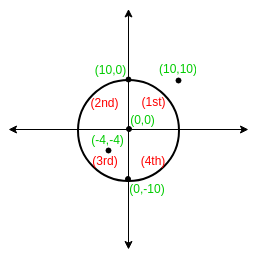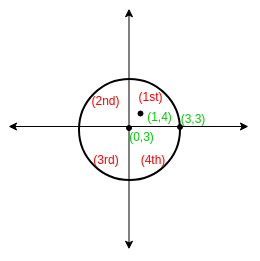# Finding Quadrant of a Coordinate with respect to a Circle

• Difficulty Level : Medium
• Last Updated : 13 May, 2021

Given the radius and coordinates of the Centre of a circle. Find the quadrant in which another given coordinate (X, Y) lies with respect to the Centre of the circle if the point lies inside the circle. Else print an error “Lies outside the circle”.
If the point lies at the Centre of circle output 0 or if the point lies on any of the axes and inside the circle output the next quadrant in anti-clock direction.

Examples:

Attention reader! Don’t stop learning now. Get hold of all the important mathematical concepts for competitive programming with the Essential Maths for CP Course at a student-friendly price. To complete your preparation from learning a language to DS Algo and many more,  please refer Complete Interview Preparation Course.

Input : Centre = (0, 0), Radius = 10
(X, Y) = (10, 10)
Output : Lies Outside the CircleInput : Centre = (0, 3), Radius = 2
(X, Y) = (1, 4)Approach:
Let center be (x’, y’)
Equation of circle is– (Eq. 1)
According to this equation,
Ifpoint (x, y) lies outside of circle
Ifpoint (x, y) lies on the circle
Ifpoint (x, y) lies inside of circle
To check position of point with respect to circle:-

```1. Put given coordinates in equation 1.
2. If it is greater than 0 coordinate lies outside circle.
3. If point lies inside circle find the quadrant within the circle. Check the point
with respect to centre of circle. ```

Below is the implementation of above idea :

## C++

 `// CPP Program to find the quadrant of``// a given coordinate with respect to the``// centre of a circle``#include ` `using` `namespace` `std;` `// Thus function returns the quadrant number``int` `getQuadrant(``int` `X, ``int` `Y, ``int` `R, ``int` `PX, ``int` `PY)``{``    ``// Coincides with center``    ``if` `(PX == X && PY == Y)``        ``return` `0;` `    ``int` `val = ``pow``((PX - X), 2) + ``pow``((PY - Y), 2);` `    ``// Outside circle``    ``if` `(val > ``pow``(R, 2))``        ``return` `-1;` `    ``// 1st quadrant``    ``if` `(PX > X && PY >= Y)``        ``return` `1;` `    ``// 2nd quadrant``    ``if` `(PX <= X && PY > Y)``        ``return` `2;` `    ``// 3rd quadrant``    ``if` `(PX < X && PY <= Y)``        ``return` `3;` `    ``// 4th quadrant``    ``if` `(PX >= X && PY < Y)``        ``return` `4;``}` `// Driver Code``int` `main()``{``    ``// Coordinates of centre``    ``int` `X = 0, Y = 3;` `    ``// Radius of circle``    ``int` `R = 2;` `    ``// Coordinates of the given point``    ``int` `PX = 1, PY = 4;` `    ``int` `ans = getQuadrant(X, Y, R, PX, PY);``    ``if` `(ans == -1)``        ``cout << ``"Lies Outside the circle"` `<< endl;``    ``else` `if` `(ans == 0)``        ``cout << ``"Coincides with centre"` `<< endl;``    ``else``        ``cout << ans << ``" Quadrant"` `<< endl;``    ``return` `0;``}`

## Java

 `// Java Program to find the quadrant of``// a given coordinate with respect to the``// centre of a circle``import` `java.io.*;``class` `GFG {` `// Thus function returns``// the quadrant number``static` `int` `getQuadrant(``int` `X, ``int` `Y,``                       ``int` `R, ``int` `PX,``                       ``int` `PY)``{``    ` `    ``// Coincides with center``    ``if` `(PX == X && PY == Y)``        ``return` `0``;` `    ``int` `val = (``int``)Math.pow((PX - X), ``2``) +``              ``(``int``)Math.pow((PY - Y), ``2``);` `    ``// Outside circle``    ``if` `(val > Math.pow(R, ``2``))``        ``return` `-``1``;` `    ``// 1st quadrant``    ``if` `(PX > X && PY >= Y)``        ``return` `1``;` `    ``// 2nd quadrant``    ``if` `(PX <= X && PY > Y)``        ``return` `2``;` `    ``// 3rd quadrant``    ``if` `(PX < X && PY <= Y)``        ``return` `3``;` `    ``// 4th quadrant``    ``if` `(PX >= X && PY < Y)``        ``return` `4``;``        ``return` `0``;``}` `    ``// Driver Code``    ``public` `static` `void` `main (String[] args)``    ``{``        ` `        ``// Coordinates of centre``        ``int` `X = ``0``, Y = ``3``;``    ` `        ``// Radius of circle``        ``int` `R = ``2``;``    ` `        ``// Coordinates of the given point``        ``int` `PX = ``1``, PY = ``4``;``    ` `        ``int` `ans = getQuadrant(X, Y, R, PX, PY);``        ``if` `(ans == -``1``)``            ``System.out.println( ``"Lies Outside the circle"``);``        ``else` `if` `(ans == ``0``)``            ``System.out.println( ``"Coincides with centre"``);``        ``else``            ``System.out.println( ans +``" Quadrant"``);``    ``}``}` `// This code is contributed by anuj_67.`

## Python3

 `# Python3 Program to find the``# quadrant of a given coordinate``# w.rt. the centre of a circle``import` `math` `# Thus function returns the``# quadrant number``def` `getQuadrant(X, Y, R, PX, PY):``    ` `    ``# Coincides with center``    ``if` `(PX ``=``=` `X ``and` `PY ``=``=` `Y):``        ``return` `0``;` `    ``val ``=` `(math.``pow``((PX ``-` `X), ``2``) ``+``           ``math.``pow``((PY ``-` `Y), ``2``));` `    ``# Outside circle``    ``if` `(val > ``pow``(R, ``2``)):``        ``return` `-``1``;` `    ``# 1st quadrant``    ``if` `(PX > X ``and` `PY >``=` `Y):``        ``return` `1``;` `    ``# 2nd quadrant``    ``if` `(PX <``=` `X ``and` `PY > Y):``        ``return` `2``;` `    ``# 3rd quadrant``    ``if` `(PX < X ``and` `PY <``=` `Y):``        ``return` `3``;` `    ``# 4th quadrant``    ``if` `(PX >``=` `X ``and` `PY < Y):``        ``return` `4``;` `# Driver Code``# Coordinates of centre``X ``=` `0``;``Y ``=` `3``;` `# Radius of circle``R ``=` `2``;` `# Coordinates of the given po``PX ``=` `1``;``PY ``=` `4``;` `ans ``=` `getQuadrant(X, Y, R, PX, PY);``if` `(ans ``=``=` `-``1``) : ``print``(``"Lies Outside the circle"``);``elif` `(ans ``=``=` `0``) : ``print``(``"Coincides with centre"``);``else``:``print``(ans, ``"Quadrant"``);` `# This code is contributed by mits`

## C#

 `// C# Program to find the quadrant of``// a given coordinate with respect to``// the centre of a circle``using` `System;` `class` `GFG {` `    ``// Thus function returns``    ``// the quadrant number``    ``static` `int` `getQuadrant(``int` `X, ``int` `Y,``                  ``int` `R, ``int` `PX, ``int` `PY)``    ``{``        ` `        ``// Coincides with center``        ``if` `(PX == X && PY == Y)``            ``return` `0;``    ` `        ``int` `val = (``int``)Math.Pow((PX - X), 2)``               ``+ (``int``)Math.Pow((PY - Y), 2);``    ` `        ``// Outside circle``        ``if` `(val > Math.Pow(R, 2))``            ``return` `-1;``    ` `        ``// 1st quadrant``        ``if` `(PX > X && PY >= Y)``            ``return` `1;``    ` `        ``// 2nd quadrant``        ``if` `(PX <= X && PY > Y)``            ``return` `2;``    ` `        ``// 3rd quadrant``        ``if` `(PX < X && PY <= Y)``            ``return` `3;``    ` `        ``// 4th quadrant``        ``if` `(PX >= X && PY < Y)``            ``return` `4;``            ``return` `0;``    ``}` `    ``// Driver Code``    ``public` `static` `void` `Main ()``    ``{``    ` `        ``// Coordinates of centre``        ``int` `X = 0, Y = 3;``    ` `        ``// Radius of circle``        ``int` `R = 2;``    ` `        ``// Coordinates of the given point``        ``int` `PX = 1, PY = 4;``    ` `        ``int` `ans =``             ``getQuadrant(X, Y, R, PX, PY);``        ``if` `(ans == -1)``            ``Console.WriteLine( ``"Lies Outside"``                            ``+ ``" the circle"``);``        ``else` `if` `(ans == 0)``            ``Console.WriteLine( ``"Coincides "``                          ``+ ``"with centre"``);``        ``else``            ``Console.WriteLine( ans +``                               ``" Quadrant"``);``    ``}``}` `// This code is contributed by anuj_67.`

## PHP

 ` pow(``\$R``, 2))``        ``return` `-1;` `    ``// 1st quadrant``    ``if` `(``\$PX` `> ``\$X` `and` `\$PY` `>= ``\$Y``)``        ``return` `1;` `    ``// 2nd quadrant``    ``if` `(``\$PX` `<= ``\$X` `and` `\$PY` `> ``\$Y``)``        ``return` `2;` `    ``// 3rd quadrant``    ``if` `(``\$PX` `< ``\$X` `and` `\$PY` `<= ``\$Y``)``        ``return` `3;` `    ``// 4th quadrant``    ``if` `(``\$PX` `>= ``\$X` `and` `\$PY` `< ``\$Y``)``        ``return` `4;``}` `    ``// Driver Code``    ``// Coordinates of centre``    ``\$X` `= 0; ``\$Y` `= 3;` `    ``// Radius of circle``    ``\$R` `= 2;` `    ``// Coordinates of the given po\$``    ``\$PX` `= 1;``    ``\$PY` `= 4;` `    ``\$ans` `= getQuadrant(``\$X``, ``\$Y``, ``\$R``,``                         ``\$PX``, ``\$PY``);``    ``if` `(``\$ans` `== -1)``        ``echo` `"Lies Outside the circle"` `;``    ``else` `if` `(``\$ans` `== 0)``        ``echo` `"Coincides with centre"` `;``    ``else``        ``echo` `\$ans` `, ``" Quadrant"` `;` `// This code is contributed by anuj_67.``?>`

## Javascript

 ``

Output:

`1 Quadrant`

My Personal Notes arrow_drop_up